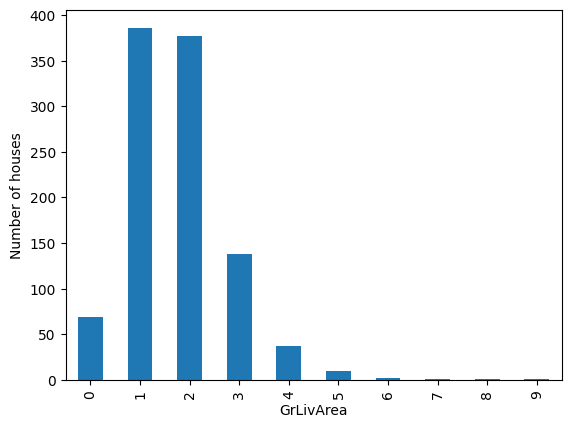# EqualWidthDiscretiser#

The `EqualWidthDiscretiser()` sorts the variable values into contiguous intervals of equal size. The size of the interval is calculated as:

( max(X) - min(X) ) / bins

where bins, which is the number of intervals, should be determined by the user. The interval limits are determined using `pandas.cut()`.

A note on number of intervals

Common values are 5 and 10. Note that if the variable is highly skewed or not continuous smaller intervals maybe required. Otherwise, the transformer will introduce np.nan.

The `EqualWidthDiscretiser()` works only with numerical variables. A list of variables to discretise can be indicated, or the discretiser will automatically select all numerical variables in the train set.

Example

Let’s look at an example using the House Prices Dataset (more details about the dataset here).

Let’s load the house prices dataset and separate it into train and test sets:

```import numpy as np
import pandas as pd
import matplotlib.pyplot as plt
from sklearn.model_selection import train_test_split

from feature_engine.discretisation import EqualWidthDiscretiser

# Separate into train and test sets
X_train, X_test, y_train, y_test =  train_test_split(
data.drop(['Id', 'SalePrice'], axis=1),
data['SalePrice'], test_size=0.3, random_state=0)
```

Now we want to discretise the 2 variables indicated below into 10 intervals of equal width:

```# set up the discretisation transformer
disc = EqualWidthDiscretiser(bins=10, variables=['LotArea', 'GrLivArea'])

# fit the transformer
disc.fit(X_train)
```

With `fit()` the transformer learns the boundaries of each interval. Then, we can go ahead and sort the values into the intervals:

```# transform the data
train_t= disc.transform(X_train)
test_t= disc.transform(X_test)
```

The `binner_dict_` stores the interval limits identified for each variable.

```disc.binner_dict_
```
```'LotArea': [-inf,
22694.5,
44089.0,
65483.5,
86878.0,
108272.5,
129667.0,
151061.5,
172456.0,
193850.5,
inf],
'GrLivArea': [-inf,
768.2,
1202.4,
1636.6,
2070.8,
2505.0,
2939.2,
3373.4,
3807.6,
4241.799999999999,
inf]}
```

With equal width discretisation, each bin does not necessarily contain the same number of observations.

```train_t.groupby('GrLivArea')['GrLivArea'].count().plot.bar()
plt.ylabel('Number of houses')
```

We can see below that the intervals contain different number of observations.Discretisation plus encoding

If we return the interval values as integers, the discretiser has the option to return the transformed variable as integer or as object. Why would we want the transformed variables as object?

Categorical encoders in Feature-engine are designed to work with variables of type object by default. Thus, if you wish to encode the returned bins further, say to try and obtain monotonic relationships between the variable and the target, you can do so seamlessly by setting `return_object` to True. You can find an example of how to use this functionality here.

## More details#

Check also for more details on how to use this transformer: